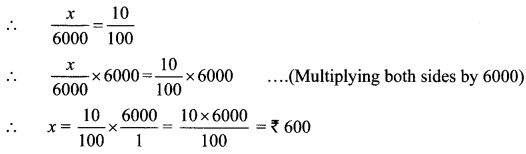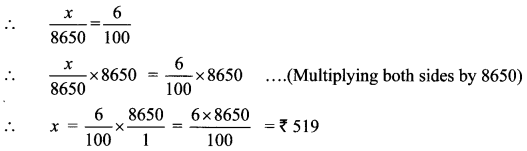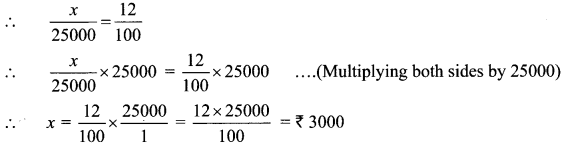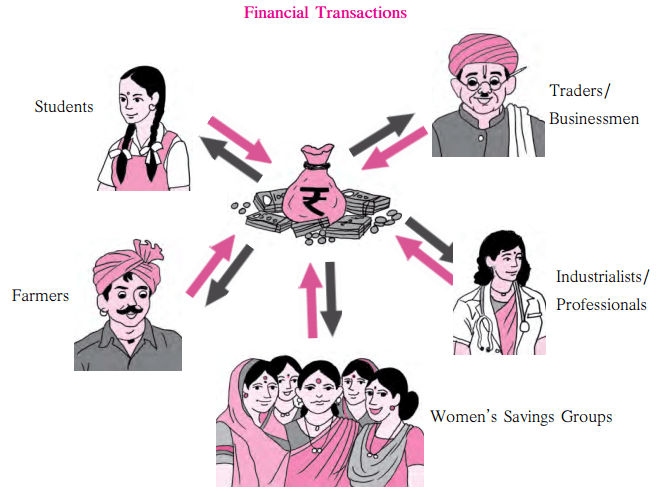# Maharashtra Board Class 6 Maths Solutions Chapter 14 Banks and Simple Interest Practice Set 35

## Maharashtra State Board Class 6 Maths Solutions Chapter 14 Banks and Simple Interest Practice Set 35

Question 1.
At a rate of 10 p.c.p.a., what would be the interest for one year on Rs 6000?
Solution:
Principal Amount = Rs 6000
Rate of Interest = 10 p.c.p.a.
Let interest on principal Rs 6000 be Rs x.
By taking ratio of the interest to the principal for both, we obtain an equation x 10∴ The interest for one year is Rs 600.

Question 2.
Mahesh deposited Rs 8650 in a bank at a rate of 6 p.c.p.a. How much money will he get at the end of the year in all?
Solution:
Principal Amount = Rs 8650
Rate of interest = 6 p.c.p.a.
Let interest on principal Rs 8650 be Rs x
By taking ratio of the interest to the principal for both, we obtain an equation∴ Amount received at the end of the year = Principal amount + Interest
= Rs 8650 + Rs 519
= Rs 9169
∴ Mahesh will get Rs 9169 at the end of the year.

Question 3.
Ahmad Chacha borrowed Rs 25,000 at 12 p.c.p.a. for a year. What amount will he have to return to the bank at the end of the year?
Solution:
Principal Amount = Rs 25,000
Rate of interest = 12 p.c.p.a.
Let interest on principal Rs 25,000 be Rs x
By taking ratio of the interest to the principal for both, we obtain an equationAmount to be returned to the bank at the end of the year = Principal Amount + Interest
= Rs 25,000 + Rs 3,000
= Rs 28,000
Ahmad Chacha has to return Rs 28,000 to the bank at the end of the year.

Question 4.
Kisanrao wanted to make a pond in his field. He borrowed Rs 35,250 from a bank at an interest rate of 6 p.c.p.a. How much interest will he have to pay to the bank at the end of the year?
Solution:
Principal Amount = Rs 35,250
Rate of interest = 6 p.c.p.a.
Let interest on principal Rs 35,250 be Rs x
By taking ratio of the interest to the principal for both, we obtain an equation∴ Kisanrao will have to pay an interest of Rs 2115 to the bank at the end of the year.

#### Maharashtra Board Class 6 Maths Chapter 14 Banks and Simple Interest Practice Set 35 Intext Questions and Activities

Question 1.
Study the figure given below and answer the following questions (Textbook pg. no. 74)

1. In the above picture, who are the people shown to be using bank services?
2. What does the symbol on the bag in the centre stand for?
3. What do the arrows in the given picture tell you?Solution:

1. Students, farmers, Women’s savings groups, industrialists / professionals and traders / businessmen are shown to be using bank services.
2. The symbol on the bag in the center stands for rupees.
3. The arrows tell us about the monetary transactions taking place. In simple words, it explains the give and take relationship.

Question 2.
Visit the Bank (Textbook pg. no. 74)

• Teachers should organise a visit to a bank. Encourage the children to obtain some preliminary information about banks.
• Help them to fill some bank forms and slips for withdrawals and deposits.
• If there is no bank nearby, teachers could obtain specimen forms and get the children to fill them in class.
• Give a demonstration of banking transactions by setting up a mock bank in the school.
• Invite participation of parents who work in banks or other bank employees to give the children more detailed information about banking.

Solution:
( Student should attempt this activity with the help of their teacher/parents.)